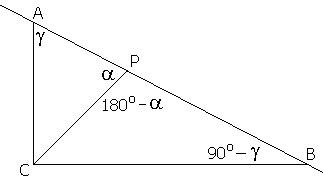# Point on Bisector in Right Angle

A straight line drawn through point P on the bisector of a right angle, intercepts on the sides of the angle segments of lengths a and b. Prove that the sum 1/a + 1/b is independent of the line but is the function solely of point P.

Proof

Copyright © 1996-2018 Alexander Bogomolny

A straight line drawn through point P on the bisector of a right angle, intercepts on the sides of the angle segments of lengths a and b. Prove that the sum 1/a + 1/b is independent of the line but is the function solely of point P.

The statement is quite simple. We'll give two proofs, trigonometric and synthetic.

Let the right angle be C and the line through point P intersect the sides of the angle in points A and B so that a = BC and b = AC.

### Proof 1Introduce α = ∠CPA and γ = &CAP. In ΔACP, by the law of sines

sinγ/CP = sinα/b.

In ΔBCP, sin(∠CBP) = sin(90° - γ) = cosγ and sin(∠BPC) = sin(180° - α) = sinα. By the law of sines,

cosγ/CP = sinα/a.

It follows that

1/a + 1/b = (sinγ + cosγ)/sinα/CP.

In ΔACP, α + γ = 135°. Since sin(135°) = -cos(135°) = 2/2, we obtain by the addition/subtraction formulas for sine

sinα = sin(135° - γ) = 2/2(sinγ + cosγ),

from which

1/a + 1/b = 2/CP,

independent of either γ or α.

The expression CP/2 relates a diagonal of a square to its side. This suggests

### Proof 2Drop perpendiculars KP and LP from P to the sides AC and BC, respectively. Since CP is the angle bisector of the right angle ACB, CKPL is a square. Let its side be h. Triangles AKP and PLB are similar, leading to a proportion

AK/KP = LP/BL or (b - h) / h = h / (a - h),

which we modify to

ab - h(a + b) + h² = h²,

and next to

ab = h(a + b).

This, after the division by abh, yields the required

1/a + 1/b = 1/h,

with h only dependent on the position of point P on the angle bisector.

### References

1. V. V. Prasolov, Problems in Planimetry I, Nauka, 1986 (in Russian), 1.17### Related materialRead more...

• Angle Bisectors In Rectangle
• Segment Trisection Induced by Parallels to Medians
• The Nature of Pi
• Parallelogram and Similar Triangles
• Two Triples of Similar Triangles
• Three Similar Triangles
• Similar Triangles on Sides and Diagonals of a Quadrilateral
•Copyright © 1996-2018 Alexander Bogomolny

 65270873